# Mean State

Period Mean (original grids) [K]
Model Period Mean (intersection) [K]
Model Period Mean (complement) [K]
Benchmark Period Mean (intersection) [K]
Benchmark Period Mean (complement) [K]
Bias [K]
RMSE [K]
Phase Shift [months]
Bias Score 
RMSE Score 
Seasonal Cycle Score 
Spatial Distribution Score 
Interannual Variability Score 
Overall Score 
Benchmark [-] 10.8
CLM4 [-] 9.47 9.51 5.64 10.9 8.01 -1.54 2.21 1.11 0.407 0.378 0.826 0.992 0.547 0.588
CLM4.5 [-] 9.17 9.18 5.70 10.9 8.01 -1.85 2.43 1.10 0.355 0.381 0.829 0.980 0.539 0.578
CLM5 [-] 8.65 8.67 5.39 10.9 8.01 -2.36 2.75 1.14 0.215 0.376 0.827 0.978 0.545 0.553
Period Mean (original grids) [K]
Model Period Mean (intersection) [K]
Model Period Mean (complement) [K]
Benchmark Period Mean (intersection) [K]
Benchmark Period Mean (complement) [K]
Bias [K]
RMSE [K]
Phase Shift [months]
Bias Score 
RMSE Score 
Seasonal Cycle Score 
Spatial Distribution Score 
Interannual Variability Score 
Overall Score 
Benchmark [-] 13.8
CLM4 [-] 12.9 13.0 7.78 13.8 11.0 -0.867 3.17 0.859 0.592 0.437 0.911 0.848 0.572 0.633
CLM4.5 [-] 12.3 12.4 7.59 13.8 11.0 -1.44 3.50 0.910 0.516 0.438 0.905 0.751 0.556 0.601
CLM5 [-] 12.0 12.1 7.63 13.8 11.0 -1.70 3.48 0.958 0.513 0.440 0.903 0.818 0.532 0.608
Period Mean (original grids) [K]
Model Period Mean (intersection) [K]
Model Period Mean (complement) [K]
Benchmark Period Mean (intersection) [K]
Benchmark Period Mean (complement) [K]
Bias [K]
RMSE [K]
Phase Shift [months]
Bias Score 
RMSE Score 
Seasonal Cycle Score 
Spatial Distribution Score 
Interannual Variability Score 
Overall Score 
Benchmark [-] 12.8
CLM4 [-] 11.8 11.9 5.60 12.8 9.07 -1.20 2.42 0.750 0.510 0.438 0.905 0.933 0.656 0.647
CLM4.5 [-] 11.3 11.4 5.72 12.8 9.07 -1.68 2.69 0.763 0.423 0.437 0.905 0.940 0.656 0.633
CLM5 [-] 11.2 11.3 5.50 12.8 9.07 -1.80 2.71 0.759 0.415 0.450 0.907 0.937 0.651 0.635
Period Mean (original grids) [K]
Model Period Mean (intersection) [K]
Model Period Mean (complement) [K]
Benchmark Period Mean (intersection) [K]
Benchmark Period Mean (complement) [K]
Bias [K]
RMSE [K]
Phase Shift [months]
Bias Score 
RMSE Score 
Seasonal Cycle Score 
Spatial Distribution Score 
Interannual Variability Score 
Overall Score 
Benchmark [-] 9.39
CLM4 [-] 7.95 8.03 5.62 9.41 7.83 -1.60 2.96 0.780 0.474 0.315 0.923 0.984 0.824 0.639
CLM4.5 [-] 7.98 8.06 5.64 9.41 7.83 -1.57 2.94 0.783 0.506 0.319 0.920 0.994 0.836 0.649
CLM5 [-] 6.86 6.93 4.62 9.41 7.83 -2.70 3.34 0.929 0.282 0.377 0.890 0.974 0.786 0.615
Period Mean (original grids) [K]
Model Period Mean (intersection) [K]
Model Period Mean (complement) [K]
Benchmark Period Mean (intersection) [K]
Benchmark Period Mean (complement) [K]
Bias [K]
RMSE [K]
Phase Shift [months]
Bias Score 
RMSE Score 
Seasonal Cycle Score 
Spatial Distribution Score 
Interannual Variability Score 
Overall Score 
Benchmark [-] 9.53
CLM4 [-] 5.01 5.15 3.62 9.60 8.86 -4.86 4.92 1.63 0.00742 0.390 0.765 0.775 0.651 0.497
CLM4.5 [-] 5.09 5.22 3.76 9.60 8.86 -4.77 4.82 1.61 0.00783 0.396 0.767 0.761 0.650 0.496
CLM5 [-] 4.24 4.35 3.16 9.60 8.86 -5.58 5.62 1.57 0.00240 0.397 0.772 0.590 0.635 0.466
Period Mean (original grids) [K]
Model Period Mean (intersection) [K]
Model Period Mean (complement) [K]
Benchmark Period Mean (intersection) [K]
Benchmark Period Mean (complement) [K]
Bias [K]
RMSE [K]
Phase Shift [months]
Bias Score 
RMSE Score 
Seasonal Cycle Score 
Spatial Distribution Score 
Interannual Variability Score 
Overall Score 
Benchmark [-] 10.7
CLM4 [-] 10.9 10.9 5.67 10.7 8.60 0.205 2.34 1.82 0.569 0.423 0.715 0.934 0.833 0.649
CLM4.5 [-] 10.1 10.2 5.70 10.7 8.60 -0.546 2.54 1.43 0.515 0.429 0.791 0.873 0.833 0.645
CLM5 [-] 8.39 8.41 4.38 10.7 8.60 -2.31 2.99 2.53 0.392 0.439 0.590 0.729 0.766 0.559
Period Mean (original grids) [K]
Model Period Mean (intersection) [K]
Model Period Mean (complement) [K]
Benchmark Period Mean (intersection) [K]
Benchmark Period Mean (complement) [K]
Bias [K]
RMSE [K]
Phase Shift [months]
Bias Score 
RMSE Score 
Seasonal Cycle Score 
Spatial Distribution Score 
Interannual Variability Score 
Overall Score 
Benchmark [-] 11.7
CLM4 [-] 11.8 11.9 5.74 11.8 9.57 -0.115 2.69 2.02 0.499 0.364 0.681 0.992 0.855 0.626
CLM4.5 [-] 11.3 11.4 5.52 11.8 9.57 -0.595 2.75 2.08 0.479 0.359 0.666 0.984 0.857 0.618
CLM5 [-] 10.4 10.4 4.64 11.8 9.57 -1.59 2.88 2.21 0.433 0.373 0.646 0.992 0.818 0.606
Period Mean (original grids) [K]
Model Period Mean (intersection) [K]
Model Period Mean (complement) [K]
Benchmark Period Mean (intersection) [K]
Benchmark Period Mean (complement) [K]
Bias [K]
RMSE [K]
Phase Shift [months]
Bias Score 
RMSE Score 
Seasonal Cycle Score 
Spatial Distribution Score 
Interannual Variability Score 
Overall Score 
Benchmark [-] 11.1
CLM4 [-] 11.1 11.2 4.77 11.2 8.50 -0.568 2.82 1.53 0.503 0.523 0.779 0.753 0.727 0.635
CLM4.5 [-] 10.6 10.8 4.84 11.2 8.50 -0.949 2.73 1.47 0.516 0.530 0.791 0.800 0.727 0.649
CLM5 [-] 10.2 10.3 4.49 11.2 8.50 -1.39 2.71 1.53 0.530 0.530 0.782 0.802 0.714 0.648
Period Mean (original grids) [K]
Model Period Mean (intersection) [K]
Model Period Mean (complement) [K]
Benchmark Period Mean (intersection) [K]
Benchmark Period Mean (complement) [K]
Bias [K]
RMSE [K]
Phase Shift [months]
Bias Score 
RMSE Score 
Seasonal Cycle Score 
Spatial Distribution Score 
Interannual Variability Score 
Overall Score 
Benchmark [-] 8.50
CLM4 [-] 7.30 7.38 5.39 8.51 7.45 -1.24 2.71 1.21 0.510 0.321 0.823 0.993 0.784 0.626
CLM4.5 [-] 7.16 7.24 5.32 8.51 7.45 -1.37 2.83 1.21 0.492 0.313 0.819 0.989 0.797 0.620
CLM5 [-] 6.35 6.41 4.48 8.51 7.45 -2.21 2.94 2.13 0.352 0.378 0.642 0.981 0.759 0.582
Period Mean (original grids) [K]
Model Period Mean (intersection) [K]
Model Period Mean (complement) [K]
Benchmark Period Mean (intersection) [K]
Benchmark Period Mean (complement) [K]
Bias [K]
RMSE [K]
Phase Shift [months]
Bias Score 
RMSE Score 
Seasonal Cycle Score 
Spatial Distribution Score 
Interannual Variability Score 
Overall Score 
Benchmark [-] 13.7
CLM4 [-] 12.2 12.4 5.24 13.8 10.4 -1.90 2.90 0.757 0.437 0.473 0.927 0.721 0.822 0.642
CLM4.5 [-] 12.2 12.3 5.39 13.8 10.4 -1.96 2.92 0.758 0.436 0.472 0.924 0.732 0.826 0.644
CLM5 [-] 11.8 11.9 4.97 13.8 10.4 -2.36 3.15 0.844 0.415 0.475 0.920 0.702 0.808 0.632
Period Mean (original grids) [K]
Model Period Mean (intersection) [K]
Model Period Mean (complement) [K]
Benchmark Period Mean (intersection) [K]
Benchmark Period Mean (complement) [K]
Bias [K]
RMSE [K]
Phase Shift [months]
Bias Score 
RMSE Score 
Seasonal Cycle Score 
Spatial Distribution Score 
Interannual Variability Score 
Overall Score 
Benchmark [-] 8.57
CLM4 [-] 8.16 8.23 6.00 8.60 7.69 -0.700 2.11 1.27 0.560 0.506 0.824 0.916 0.822 0.689
CLM4.5 [-] 8.26 8.32 6.17 8.60 7.69 -0.588 2.04 1.35 0.579 0.504 0.814 0.959 0.813 0.695
CLM5 [-] 6.90 6.96 4.96 8.60 7.69 -1.92 2.42 1.09 0.429 0.565 0.867 0.978 0.747 0.692
Period Mean (original grids) [K]
Model Period Mean (intersection) [K]
Model Period Mean (complement) [K]
Benchmark Period Mean (intersection) [K]
Benchmark Period Mean (complement) [K]
Bias [K]
RMSE [K]
Phase Shift [months]
Bias Score 
RMSE Score 
Seasonal Cycle Score 
Spatial Distribution Score 
Interannual Variability Score 
Overall Score 
Benchmark [-] 11.6
CLM4 [-] 10.5 11.1 5.67 11.8 7.98 -0.970 2.92 1.29 0.440 0.406 0.815 0.952 0.716 0.623
CLM4.5 [-] 10.4 10.8 6.83 11.8 7.98 -1.27 2.96 1.28 0.424 0.405 0.818 0.971 0.717 0.623
CLM5 [-] 9.62 10.0 6.09 11.8 7.98 -2.04 3.23 1.45 0.350 0.417 0.789 0.962 0.689 0.604
Period Mean (original grids) [K]
Model Period Mean (intersection) [K]
Model Period Mean (complement) [K]
Benchmark Period Mean (intersection) [K]
Benchmark Period Mean (complement) [K]
Bias [K]
RMSE [K]
Phase Shift [months]
Bias Score 
RMSE Score 
Seasonal Cycle Score 
Spatial Distribution Score 
Interannual Variability Score 
Overall Score 
Benchmark [-] 14.0
CLM4 [-] 15.8 15.8 8.91 14.0 11.1 1.52 3.13 1.21 0.345 0.329 0.837 0.792 0.644 0.546
CLM4.5 [-] 15.4 15.5 8.83 14.0 11.1 1.19 2.92 1.22 0.370 0.332 0.834 0.832 0.651 0.558
CLM5 [-] 15.2 15.2 8.67 14.0 11.1 0.929 2.89 1.25 0.379 0.324 0.832 0.834 0.628 0.553
Period Mean (original grids) [K]
Model Period Mean (intersection) [K]
Model Period Mean (complement) [K]
Benchmark Period Mean (intersection) [K]
Benchmark Period Mean (complement) [K]
Bias [K]
RMSE [K]
Phase Shift [months]
Bias Score 
RMSE Score 
Seasonal Cycle Score 
Spatial Distribution Score 
Interannual Variability Score 
Overall Score 
Benchmark [-] 8.40
CLM4 [-] 8.27 8.36 5.80 8.41 7.44 -0.321 2.68 0.738 0.597 0.261 0.905 0.969 0.815 0.635
CLM4.5 [-] 7.97 8.04 5.79 8.41 7.44 -0.609 2.61 0.699 0.624 0.260 0.916 0.992 0.825 0.646
CLM5 [-] 6.72 6.79 4.87 8.41 7.44 -1.83 2.79 1.03 0.399 0.324 0.855 0.960 0.799 0.610
Period Mean (original grids) [K]
Model Period Mean (intersection) [K]
Model Period Mean (complement) [K]
Benchmark Period Mean (intersection) [K]
Benchmark Period Mean (complement) [K]
Bias [K]
RMSE [K]
Phase Shift [months]
Bias Score 
RMSE Score 
Seasonal Cycle Score 
Spatial Distribution Score 
Interannual Variability Score 
Overall Score 
Benchmark [-] 13.3
CLM4 [-] 9.01 9.13 6.11 13.4 10.3 -5.05 5.40 1.04 0.142 0.450 0.871 0.893 0.637 0.574
CLM4.5 [-] 9.04 9.15 6.33 13.4 10.3 -4.97 5.31 1.13 0.133 0.442 0.856 0.931 0.647 0.575
CLM5 [-] 8.60 8.70 6.04 13.4 10.3 -5.41 5.73 1.24 0.114 0.439 0.838 0.917 0.633 0.564
Period Mean (original grids) [K]
Model Period Mean (intersection) [K]
Model Period Mean (complement) [K]
Benchmark Period Mean (intersection) [K]
Benchmark Period Mean (complement) [K]
Bias [K]
RMSE [K]
Phase Shift [months]
Bias Score 
RMSE Score 
Seasonal Cycle Score 
Spatial Distribution Score 
Interannual Variability Score 
Overall Score 
Benchmark [-] 12.2
CLM4 [-] 10.3 10.3 6.10 12.2 9.97 -2.03 2.55 2.29 0.369 0.454 0.627 0.855 0.741 0.583
CLM4.5 [-] 9.93 9.95 6.20 12.2 9.97 -2.40 2.84 2.49 0.296 0.445 0.589 0.891 0.744 0.568
CLM5 [-] 8.63 8.63 5.31 12.2 9.97 -3.70 3.95 2.47 0.135 0.459 0.605 0.926 0.679 0.544
Period Mean (original grids) [K]
Model Period Mean (intersection) [K]
Model Period Mean (complement) [K]
Benchmark Period Mean (intersection) [K]
Benchmark Period Mean (complement) [K]
Bias [K]
RMSE [K]
Phase Shift [months]
Bias Score 
RMSE Score 
Seasonal Cycle Score 
Spatial Distribution Score 
Interannual Variability Score 
Overall Score 
Benchmark [-] 11.0
CLM4 [-] 11.4 11.4 6.05 11.1 7.54 0.0759 2.10 1.47 0.590 0.482 0.772 0.909 0.768 0.667
CLM4.5 [-] 10.9 10.9 6.16 11.1 7.54 -0.396 2.04 1.31 0.621 0.479 0.798 0.956 0.768 0.684
CLM5 [-] 8.72 8.72 4.69 11.1 7.54 -2.51 3.03 2.39 0.327 0.477 0.605 0.996 0.711 0.599
Period Mean (original grids) [K]
Model Period Mean (intersection) [K]
Model Period Mean (complement) [K]
Benchmark Period Mean (intersection) [K]
Benchmark Period Mean (complement) [K]
Bias [K]
RMSE [K]
Phase Shift [months]
Bias Score 
RMSE Score 
Seasonal Cycle Score 
Spatial Distribution Score 
Interannual Variability Score 
Overall Score 
Benchmark [-] 15.3
CLM4 [-] 13.8 13.7 9.03 15.3 11.3 -1.70 3.11 0.718 0.457 0.421 0.932 0.985 0.861 0.680
CLM4.5 [-] 13.3 13.3 9.49 15.3 11.3 -2.14 3.29 0.736 0.421 0.416 0.928 0.974 0.862 0.670
CLM5 [-] 12.5 12.5 8.47 15.3 11.3 -2.92 3.83 0.894 0.344 0.392 0.901 0.990 0.841 0.643
Period Mean (original grids) [K]
Model Period Mean (intersection) [K]
Model Period Mean (complement) [K]
Benchmark Period Mean (intersection) [K]
Benchmark Period Mean (complement) [K]
Bias [K]
RMSE [K]
Phase Shift [months]
Bias Score 
RMSE Score 
Seasonal Cycle Score 
Spatial Distribution Score 
Interannual Variability Score 
Overall Score 
Benchmark [-] 10.2
CLM4 [-] 8.05 8.06 4.23 10.2 9.50 -2.29 2.59 0.606 0.232 0.519 0.921 0.947 0.450 0.598
CLM4.5 [-] 8.00 8.02 4.41 10.2 9.50 -2.32 2.61 0.604 0.227 0.519 0.922 0.961 0.452 0.600
CLM5 [-] 7.43 7.44 3.96 10.2 9.50 -2.90 3.09 0.600 0.155 0.507 0.920 0.989 0.456 0.589
Period Mean (original grids) [K]
Model Period Mean (intersection) [K]
Model Period Mean (complement) [K]
Benchmark Period Mean (intersection) [K]
Benchmark Period Mean (complement) [K]
Bias [K]
RMSE [K]
Phase Shift [months]
Bias Score 
RMSE Score 
Seasonal Cycle Score 
Spatial Distribution Score 
Interannual Variability Score 
Overall Score 
Benchmark [-] 11.7
CLM4 [-] 10.1 10.2 4.92 11.7 9.16 -1.65 2.61 1.08 0.418 0.379 0.842 0.837 0.556 0.569
CLM4.5 [-] 9.75 9.84 5.14 11.7 9.16 -1.99 2.78 1.02 0.356 0.377 0.853 0.884 0.551 0.566
CLM5 [-] 9.30 9.40 4.60 11.7 9.16 -2.45 2.98 0.859 0.265 0.391 0.885 0.904 0.545 0.564
Period Mean (original grids) [K]
Model Period Mean (intersection) [K]
Model Period Mean (complement) [K]
Benchmark Period Mean (intersection) [K]
Benchmark Period Mean (complement) [K]
Bias [K]
RMSE [K]
Phase Shift [months]
Bias Score 
RMSE Score 
Seasonal Cycle Score 
Spatial Distribution Score 
Interannual Variability Score 
Overall Score 
Benchmark [-] 12.5
CLM4 [-] 11.1 11.2 7.39 12.6 7.59 -1.90 2.92 1.49 0.361 0.456 0.788 0.990 0.718 0.628
CLM4.5 [-] 10.5 10.5 7.14 12.6 7.59 -2.52 3.33 1.47 0.298 0.454 0.792 0.987 0.713 0.616
CLM5 [-] 9.91 9.92 6.60 12.6 7.59 -3.13 3.75 1.37 0.238 0.470 0.814 0.988 0.673 0.609

# Temporally integrated period mean

BENCHMARK MEAN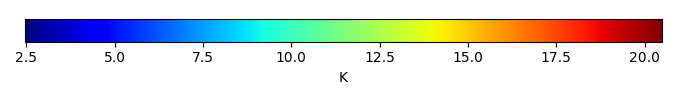MODEL MEANBIAS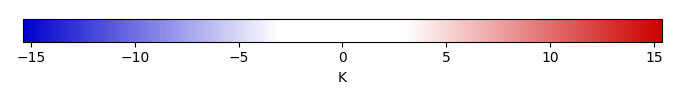BIAS SCORERMSE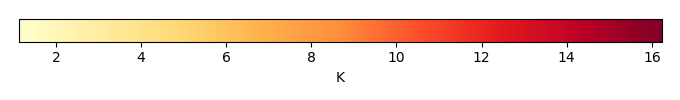RMSE SCOREBENCHMARK INTERANNUAL VARIABILITY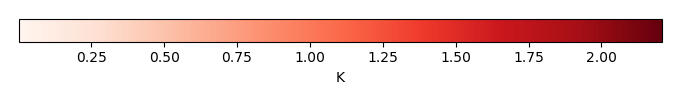MODEL INTERANNUAL VARIABILITYINTERANNUAL VARIABILITY SCOREBENCHMARK MAX MONTHMODEL MAX MONTHDIFFERENCE IN MAX MONTH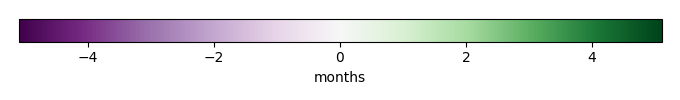SEASONAL CYCLE SCORESPATIAL TAYLOR DIAGRAMMODEL COLORS# Spatially integrated regional mean

MODEL COLORSREGIONAL MEANANNUAL CYCLEMONTHLY ANOMALYANNUAL CYCLE# All Models

BenchmarkCLM4CLM4.5CLM5# Data Information

creation_date: Fri Feb 8 13:55:45 PST 2019

history: 2018-01-15: download from https://crudata.uea.ac.uk/cru/data/hrg/cru_ts_4.01/cruts.1709081022.v4.01/ 2019-02-06: converted and created to netCDF4 for ILAMB package

original_resolution: 0.5x0.5

resolution: 0.5x0.5

original_frequency: mon

frequency: mon

references: Harris et al., 2014, Int. J. Climatol., doi:10.1002/joc.3711

institution: Original Data held at British Atmospheric Data Centre, RAL, UK.

source: Run ID = 1709081022. Data generated from:dtr.1708250000.dtb

title: CRU TS4.01 Diurnal Temperature Range

Conventions: CF-1.7